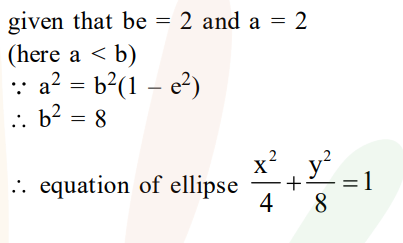# An ellipse, with foci at (0,2) and (0,-2)Question:

An ellipse, with foci at $(0,2)$ and $(0,-2)$ and minor axis of length 4 , passes through which of the following points ?

1. $(1,2 \sqrt{2})$

2. $(2, \sqrt{2})$

3. $(2,2 \sqrt{2})$

4. $(\sqrt{2}, 2)$

Correct Option: , 4

Solution: